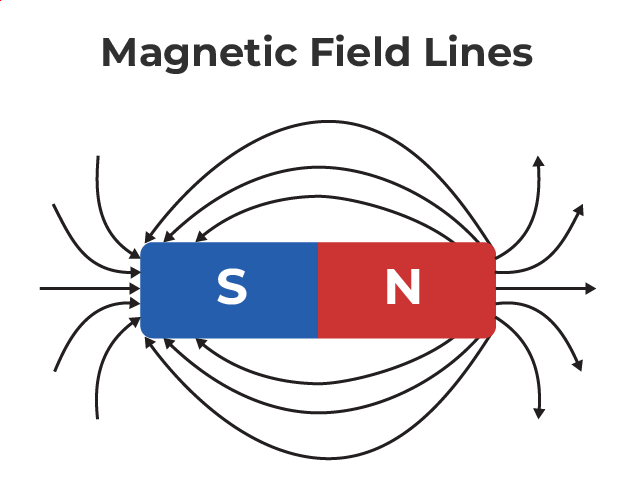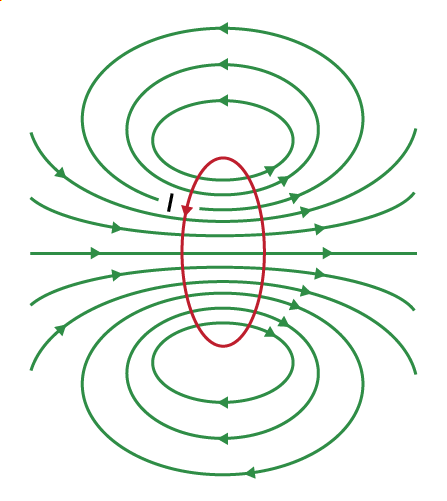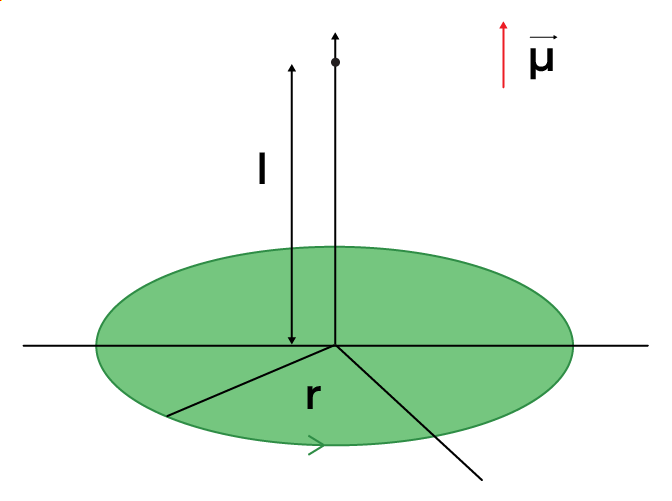Open in App
Not now

# Current Loop as a Magnetic Dipole

• Last Updated : 11 Nov, 2022

When a charge move it generates an electric field and the rate of flow of charge is the current in the electric field. This is the basic concept in Electrostatics. The magnetic effect of electric current is the other important concept related to moving electric charges. Magnetism is generated due to the flow of current. Magnetic fields exert force on the moving charges and at the same time on other magnets, all of which have moving charges. When the charges are stationary, their magnetic field doesn’t affect the magnet but when charges move, they produce magnetic fields that exert force on other magnets.

The movement of charges generates magnetism around a conductor. Generally, magnetism is a property shown by magnets and produced by moving charges, which results in objects being attracted or pushed away.

## Magnets and Magnetic fields

Moving charge creates a magnetic field and the force created in a magnetic field is called Magnetic Force. A charge is a basic property associated with the matter due to which it produces and experiences electrical and magnetic effects. A particular region in space around the magnet where the magnet has its magnetic effect is called the magnetic field of the magnet.Assume that there is a point charge q (moving with a velocity v and, located at r at given time t) in presence of both the electric field Er and the magnetic field Br. The force on the electric charge q due to both magnetic field and electric field can be written as,

F = q [Er + v × Br] ≡ EElectric + Fmagnetic

This is formula was stated by H.A.Lorentz for the force due to the electric field, based on the extensive experiments of Ampere and others. It is also called the Lorentz force.

## Characteristics of Magnets

Characteristics of a Magnets are:

• Similar poles of a magnet repel each other, when two north poles of bar magnets are brought close to each other, they move away from each other.
• Opposite poles of magnets attract each other, when two opposite poles like the north pole and south pole of two magnets, are brought close to each other, they attract each other.
• In a magnet, the north pole and south pole cannot be separated. This is because every magnet, even when broken into pieces, will create a dipole from each piece. Each piece of the magnet will always have a north pole or a south pole.
• When a magnet is freely suspended, the north pole of the magnet will point to the geographical north and the south pole of the magnet will point to the geographical south.
• The magnetic field is maximum at the poles and minimal at the middle of the magnet.

## Magnetic Dipole and Magnetic Dipole Moment

A magnetic dipole is formed by placing a magnetic north pole and south pole closer together, where the product of the magnetic pole charge and distance is constant. Andre-Marie Ampere gave the idea that a current loop can act as a magnetic dipole. This phenomenon is explained by Ampere with the help of the circular movement of current through the wire. It is similar to the magnetic dipole with the magnetic field line straight at the center of the loop. If the current flows in a circular loop inside a magnetic field it behaves as a magnetic dipole. When an electron rotates around the positively charged nuclei, it forms a magnetic dipole. The direction of the magnetic field lines is determined by the direction of current flow in the wired loop.

In a magnetic dipole, two opposite magnetic charges (bar magnet) are kept at a very short distance. Magnetic Dipole Moment is defined as the ability of a magnetic dipole to align itself with the magnetic field outside. The magnetic dipole moment is defined as the maximum amount of torque caused by magnetic force on a dipole that arises per unit value of the surrounding magnetic field in a vacuum. It is a vector quantity and its direction is from the north pole to the south pole. Its SI unit is Ampere-meter2 and its cgs unit is ergs per gauss.

The torque of the dipole can be written as,

τ = M × B

where,
τ is the torque acting on the dipole
M is the magnetic moment
B is the outside magnetic field

A magnetic dipole is the presence of both poles of a magnet, this creates a magnetic field. For example, when a bar magnet is broken into pieces, each piece will act as a magnetic dipole individually, and every piece will carry a north and a south pole.

## Current loop as a Magnetic Dipole and Its Dipole Moment

When a current passes through a circular loop it is considered a magnetic dipole. It works through the geometry of the ring which tries to align the magnetic object around it and creates a magnetic field or lines of the magnetic field. The magnetic dipole moment of a current loop is defined as the product of the current in the loop and the area of the loop.

μ = nIA

where,
n is the number of turns in the loop
I is the current in the loop
A is the area inside the loopThe SI unit of the dipole moment is ampere-meter2, and the cgs unit for the dipole moment of a current loop is erg/gauss. Where erg is the unit of energy and gauss is the unit of magnetic flux density.

## Characteristics of Magnetic Field Created by a Current Loop

Characteristics of a Magnetic Field Created by a Current Loop are:

• The lines are circular near the coil and are straight toward the center of the circular current-carrying coil.
• The magnetic force lines enter from the lower end of the coil and leave from the upper back. The lower end from which the lines seem to be entering is the south pole of the dipole. The upper end, where the lines seem to be leaving is the north pole of the dipole.
• The direction of the dipole moment of the current loop will be in a direction perpendicular to the plane of the current-carrying loop.
• The direction of the magnetic dipole is a vector with the magnetic field moving from the south pole to the north pole.

## Current Loop as a Magnetic Dipole

The circular current loop acts as a magnetic field due to the current moving in the circular direction or in a loop and its direction is determined according to the “Right-Hand Thumb” rule. The circular current loop act as a magnetic dipole as a magnetic field is generated due to the flow of charges. Hence, the formula for a circular current loop is,

μ = niπr2

where,
n is the number of turns
i is the current flowing in the circular loop
r is the radius of the loop

## Current Loop as a Magnetic Dipole Derivation

Consider a circular loop with a radius “R” on the table, which is carrying a current “i” in an anti-clockwise direction. Now, take a point P that is “l” distance apart from the center of the loop. Therefore, the magnetic field (B) at point p is given byFor simplification let us assume that the distance of the point (p) is very far from the current-carrying loop.as, R<<l

The area of the loop is given as

A = πR2

The magnetic field can be written asAs, μ = iA

The above formula for magnetic field is similar to the Electric dipole field## Solved Problems on Circular Current loop as a Magnetic Dipole

Problem 1: Two wires of the same length are shaped into a square and a circle. If they carry the same current, then the ratio of their magnetic moments is:

Solution:

The magnetic moment of a loop is given by (m) = IA

The wires have the same length,

Perimeter of a square = Circumference of a circle

Therefore, 4a = 2πr

a = πr / 2                     …(1)

The magnetic moment of the square loop is given by,

m1 = I(A1)

⇒ m1 = Iπ2r2 / 4                      [from equation (1)]      …(2)

The magnetic dipole moment of a circular loop is given by,

m2 = I(A2)

m2 = I(πr2)                               …(3)

From equations (2) and (3), we get

m1 / m2 = π / 4

Problem 2: A circular coil of 300 turns and a diameter of 14 cm carries a current of 15 A. The magnitude of the magnetic moment associated with the loop is going to be

Solution:

Given:

Number of turns (N) = 300

Radius of coil (r) = 14/2 = 7cm = 7 × 10-2 m

Current in coil (I) = 15A

Magnetic moment (M) = NI(πr2)

M = 300 × 15 × 22/7 × (7 × 10-2)2

M = 69.2 JT-1

Problem 3: A circular coil of 100 turns, each turn of radius 8 cm carries a current of 0.4 A. What is the magnitude of the magnetic field B at the center of the coil?

Solution:

Given:

Number of turns (N) = 100

radius of each turn (r) = 8cm = 8 × 10-2m

Current flowing in the coil (I) = 0.4 A

permeability of free space (μ0) = 4π × 10-7 TmA-1

The magnitude of the magnetic field at the center of the coil can be obtained by,

|B| = μ0 2πnI / 4πr

|B| = (4π × 10-7 / 4π) × (2π × 100 × 0.4 / 8 × 10-2)

|B| = 3.14 × 10-4 T

Problem 4: A long straight wire in the horizontal plane carries 50 A in the north-to-south direction. Give the magnitude and direction of B at a point 2.5 m east of the wire.

Solution:

Given:

Current in the wire (I) = 50 A

Distance of a point from the wire (r) = 2.5 m

permeability of free space (μ0) = 4π × 10-7 TmA-1

The magnitude of the magnetic field at a given point can be obtained by,

|B| = μ0 2I / 4πr

|B| = (4π × 10-7 / 4π) × (2 × 50 / 2.5)

|B| = 4 × 10-6 T

Problem 5: Two very long wires are placed parallel to each other and separated by a distance of 1m apart. If the current in both the wires is 1A, then the force per unit length on both wires will be:

Solution:

Current in both the wires Ia = Ib = 1A

distance (d) = 1m

The force per unit length is given as,

fab = fba = f = μ0IaIb / 2πd           —(1)

from equation (1),

f = (μ0×1×1) / (2π×1)

f = μ0/2π × 2/2

f = 2μ0/4π                —(2)

we know,

μ0/4π = 10-7 T – m/A        —(3)

from equations (2) and (3),

f = 2 × 10-7 N

## FAQs Circular Current Loop as a Magnetic Dipoles

Question 1: What is a magnetic dipole?

A magnetic dipole is an object where two opposite magnetic poles (north and south) of equal strength are kept at a very short distance, these dipoles can be bar magnets.

Question 2: State Characteristics of Magnetic Field Lines.

Few of the characteristics of magnetic Field Lines are:

1. The magnetic field lines form continuous and closed loops.
2. Magnetic field lines never intersect each other.
3. The strength of the magnetic field in a particular area can be determined by the number of field lines crossing per unit area. The more the number of lines, the more the strength of the magnetic field at that point.
4. The tangent drawn from any field line will give the direction of the magnetic field at that point.

Question 3: Define Magnetic Dipole Moment.

The magnetic dipole moment is defined as the maximum amount of torque generated by magnetic force on a dipole that arises per unit value of the surrounding magnetic field in a vacuum.

Question 4: What is the difference between Magnetic dipole and Magnetic dipole moment?

A magnetic north pole and a magnetic south pole separated by a short distance are termed a magnetic dipole. Magnetic dipole moments have dimensions of current times area of energy divided by magnetic flux density.

Question 5: Define the Magnetic field.